Question of The Day25-07-2022

How many cylindrical bullets can be made out of a lead sphere of 6 cm radius, if bullets have a base of 0.5 cm in radius and a height of 1 cm?

Correct Answer : b ) 1152

Explanation :

As we know that the bullets are being made from the sphere, so the total volume of bullets will be equal to the volume of the sphere.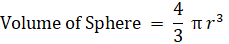Radius of sphere = 6 cm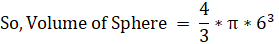And, Volume of cylindrical bullet = πr2h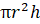Volume of cylindrical bullet = π* (0.5)2*1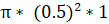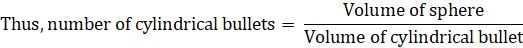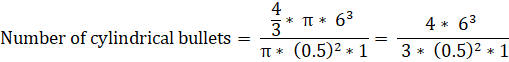Therefore, the Number of cylindrical bullets = 1152

Hence, b) is the correct answer.0